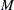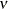The QLIM Procedure

PRIOR Statement

• PRIOR _REGRESSORS |parameter-list ~ distribution ;

The PRIOR statement specifies the prior distribution of the model parameters. You must specify a single parameter or a list of parameter, a tilde ~, and then a distribution with its parameters. Multiple PRIOR statements are allowed.

You can specify the following distributions:

NORMAL(MEAN=, VAR=)

specifies a normal distribution with parameters MEAN and VAR.

GAMMA(SHAPE=a, SCALE=b)

specifies a gamma distribution with parameters SHAPE and SCALE.

IGAMMA(SHAPE=a, SCALE=b)

specifies an inverse gamma distribution with parameters SHAPE and SCALE.

UNIFORM(MIN=m, MAX=)

specifies a uniform distribution that is defined between MIN and MAX.

BETA(SHAPE1=a, SHAPE2=b, MIN=m, MAX=)

specifies a beta distribution with parameters SHAPE1 and SHAPE2 and defined between MIN and MAX.

T(LOCATION=, DF=)

specifies a noncentral t distribution with DF degrees of freedom and location parameter equal to LOCATION.

See the section Standard Distributions for details about how to specify distributions.

You can specify the special keyword REGRESSORS to select all the parameters used in the linear regression component of the model.